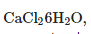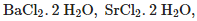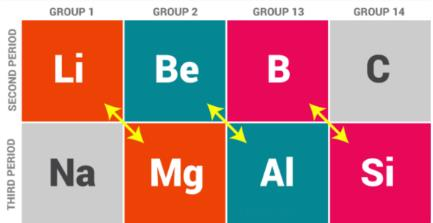Courses

# Test Level 1 : s-Block Elements

## 25 Questions MCQ Test Chemistry Class 11 | Test Level 1 : s-Block Elements

Description
This mock test of Test Level 1 : s-Block Elements for JEE helps you for every JEE entrance exam. This contains 25 Multiple Choice Questions for JEE Test Level 1 : s-Block Elements (mcq) to study with solutions a complete question bank. The solved questions answers in this Test Level 1 : s-Block Elements quiz give you a good mix of easy questions and tough questions. JEE students definitely take this Test Level 1 : s-Block Elements exercise for a better result in the exam. You can find other Test Level 1 : s-Block Elements extra questions, long questions & short questions for JEE on EduRev as well by searching above.
QUESTION: 1

### Sodium and potassium belong to the category of

Solution:

Na and K have ns1 configuration so they are alkali metal.

QUESTION: 2

### What is the oxidation state of K in KO2?

Solution:

Here KO2 is in superoxide form hence O.S. of O is -1/2 for each oxygen atom. Hence 2*1/2= 1, therefore k has +1 O.S.

QUESTION: 3

### Potassium ions are the most abundant cations. They:

Solution:

Potassium ions are the most abundant cations. They participate in the oxidation of glucose to produce ATP

QUESTION: 4

Metal carbonates decompose on heating to give metal oxide and carbondioxide. Which of the metal carbonates is most stable thermally?

Solution:

BaCO3 is very stable due to size compatability factor . A larger cation can stablises a larger anion.

QUESTION: 5

Dehydration of hydrates of halides of calcium, barium and strontium i.e.,can be achieved by heating. These become wet on keeping in air. Which of the following statements is correct about these halides?

Solution:

All of these statements are coorect as these halides absorb water and due to decrease in the hydration energy down the group tendency to from hydrates decreases.

QUESTION: 6

One of the following is highly radioactive

Solution:

Fr is radioactive.

QUESTION: 7

2M +2H2O → 2M+ + 2OH+ H2

What is M ?

Solution:

M is alkali metal.

QUESTION: 8

To acquire noble gas configuration, alkaline earth metals lose

Solution:

Alkaline Earth Metals loses 2 electron to attain noble gas configuration.

QUESTION: 9

The diagonal relationship exists between

Solution:

1st element of 1st group and the second element of 2nd group exist in diagonal relationship.Hence C is the correct answer.

QUESTION: 10

Choose the correct statements from the following.

Solution:

The correct answer is Option B
Be is not readily attacked by acids due to formation of oxide layer.
The surface of beryllium metal is covered with a thin layer of oxide that helps protect the metal from attack by air. It does not oxidize in air even at 600oC.
2Be+O2⟶2BeO

QUESTION: 11

Which one of the following orders presents the correct sequence of the increasing basic nature of the given oxides?

Solution:

While moving from left to right in periodic table basic character of oxide of elements will decrease. ∴→Increasing basic strength Al2O3<MgO<Na2O    and while descending in the group basic character of corresponding oxides increases.  ∴→Increasing basic strength Na2O<K2O   ∴ Correct Order is Al2O3 < MgO <Na2O <K2O

QUESTION: 12

Lithium has most negative E0 value,its reaction with water is less vigorous than that of sodium which has the least negative E0 value among the alkali metals. The reason is

Solution:

Li due its small size have high Lattice energy value which compensates its high ionization energy so has most negative E0 value

QUESTION: 13

Alkaline earth metals show large size of the atoms. Therefore, they have

Solution:

The low ionisation enthalpies of the alkaline earth metals is because of their strong tendency to lose electrons due to their smaller nuclear charge and comparatively larger atomic size which results in weaker forces of attraction between the valence electrons and the nucleus.

QUESTION: 14

Metals form basic hydroxides. Which of the following metal hydroxide is the least basic?

Solution:

Due to small size of Mg. Mg-O become more stronger hence tendency of OH to leave decreases thus basic character decreases.

QUESTION: 15

Several sodium compounds find use in industries. Which of the following ompounds are used for textile industry?

Solution:

Na2CO3 is used in textile industry.

QUESTION: 16

Monovalent sodium and potassium ions found in large proportions in biological fluids. They help in

Solution:

Na and K ions help in transmission of nerve impulses.

QUESTION: 17

When sodium is dissolved in liquid ammonia, a solution of deep blue colour is obtained. The colour of the solution is due to

Solution:

The color of solution is due to the presence of ammoniated electron.

QUESTION: 18

Choose the correct order of increase in electropositivity of alkali earth metals in the options given below

Solution:

Correct Answer : a

Explanation : Atomic no. of Be, Mg, Ca, Sr, Ba are 4, 12,20,38,56 respectively.

On moving down the group, the effective nuclear charge on the outermost electrons decreases. Hence Ba can readily lose electrons and will be the most electropositive element among the alkaline earth metals.

QUESTION: 19

Some of the Group 2 metal halides are covalent and soluble in organic solvents. Among the following metal halides, the one which is soluble in ethanol is

Solution:

BeCl2 is soluble in ethanol. Except for beryllium halides, all other halides of alkaline earth metals are ionic in nature. Beryllium halides are essentially covalent and soluble in organic solvents.

QUESTION: 20

Which of the following compounds are readily soluble in water?

Solution:

BeSO4 being unstable is readily soluble in water. Aslo hydration energy of Be is high due to small size.

QUESTION: 21

Divalent magnesium and calcium ions are found in large proportions in biological fluids.

Solution:

Mg2+ and Ca2+ maintains the ion balance.

QUESTION: 22

The alkali metals dissolve in liquid ammonia giving deep blue solutions. When they become diamagnetic, the solution turns into

Solution:

When the solution become diamagnetic color changes to bronze.

QUESTION: 23

Radium salts are used in

Solution:

Ra salts are used in the treatment of Cancer.

QUESTION: 24

The solubility of metal halides depends on their nature, lattice enthalpy and hydration enthalpy of the individual ions. Amongst fluorides of alkali metals,the lowest solubility of LiF in water is due to

Solution:

LiF has very high lattice energy which cannot be compensated by Hydration Energy.

QUESTION: 25

Which of the following are the correct reasons for anomalous behaviour of lithium?

Solution:

Exceptionally small size of Li is responsible for its anamolous behavior.